American Journal of Optics and Photonics
Volume 3, Issue 1, February 2015, Pages: 5-12

Performance Analysis of Coherent Optical OFDM Applied to UAV Mobile FSO Systems

Konstantinos Eleftherios Zarganis, Antonis Hatziefremidis

Department of Aircraft Technology, Technological Educational Institute of Sterea Ellada, Psahna, Greece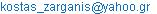(K. E. Zarganis)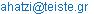(A. Hatziefremidis)

Konstantinos Eleftherios Zarganis, Antonis Hatziefremidis. Performance Analysis of Coherent Optical OFDM Applied to UAV Mobile FSO Systems. American Journal of Optics and Photonics. Vol. 3, No. 1, 2015, pp. 5-12. doi: 10.11648/j.ajop.20150301.12

Abstract: The Free Space Optical (FSO) communication links is a promising and cost-effective solution for the provision of high data rate point to point communications. Due to their huge bandwidth, license free spectrum and multipath fading mitigation, the FSO links combined with Orthogonal Frequency Division Multiplexing (OFDM) modulation technique provide an ideal communication solution between Unmanned Aerial Vehicles (UAVs) and fixed Ground Station (GS). In order to design a high performance communication link, it is important to characterize the quality of the optical channel with a proper model, considering the interesting challenges of environmental conditions, such as turbulence and visibility conditions. In this paper we are studying the performance of a mobile link among co-moving UAVs, UAV and GS at different fixed altitudes and link ranges via Optisystem simulation platform, in terms of Bit Error Rate (BER), Optical Signal to Noise Ratio (OSNR) and constellation diagrams. An extensive, comparative analysis among different FSO configurations links considering various characteristics of the OFDM modulation, link ranges, Bit Rates (BR) and the atmospherical conditions is provided.

Keywords: Mobile FSO, OFDM, UAV, BER

Contents

1. Introduction

For many years the Free Space Optical (FSO) communication system has been an interesting and promising alternative for the enormous, current telecom demands, providing a solution for broadband networks, especially in geographical areas where optical fiber deployment is not feasible .

The FSO systems have a number of advantages compared to conventional free space radio frequency systems. Cost-effective deployment, license free and high bandwidth access techniques to name a few are elements that classify these systems in the centre of attention for the new telecom technologies .

However the performance of a FSO link can be degraded by many reasons due to variable atmospheric conditions. It should also be stated the existence of multipath fading is one of the dominant factors that impoverish the functioning of such systems. For the multipath fading what can be attempted is the introduction of multiple carriers modulation as OFDM. OFDM is one of the most popular technique for broadband wireless telecommunications and its known for its increased robustness against frequency selective fading in contrast to single carrier systems, narrow-band interference and high channel efficiency [3,4]. In conclusion, all the above make OFDM an ideal candidate for FSO transmission. Despite the fact that OFDM has been extensively studied in the radio frequency domain, it is quite surprising that optical OFDM has only been reported in the last two decades . Coherent Optical OFDM (CO-OFDM) represents the ultimate performance in receiver sensitivity and spectral efficiency by overlapping subcarriers spectrum while avoiding interference via the combination of coherent detection and signal set orthogonality.

In this paper we are researching the transmission performance of the optical OFDM signals over a turbulent and non turbulent FSO mobile channel between co-moving Unmanned Aerial Vehicles (UAVs) and between UAV and Ground Station (GS) in different link configurations. The performance of the system has been evaluated for clear, hazy and foggy weather conditions and the results show that the system can provide a reliable communication link (BER value at 1e-6) for 35km, 12km and 3km respectively. The research took place in a simulating computational environment via Optisystem platform by Optiwave Systems Inc. The work in this paper is organized as follows: in section 2, a system analysis is presented. In section 3, we are introducing an extensive mathematical analysis and derive expressions for a turbulent and non turbulent environment and for various visibility conditions of the link, in order to provide an accurate mathematical expression for the estimation of the Bit Error Rate (BER) of the proposed model. In Section 4, the simulation results are presented and finally in Section 5, we report the results of this research.

2. System Analysis

The funtamental principle of CO-OFDM system aims at achieving high spectral efficiency by overlapping subcarriers spectrum, initially in the RF domain and finally in the optical domain via up conversion. A generic optical OFDM FSO system can be divided into five functional blocks [5,6]:

1) RF OFDM Transmitter, 2) RF to Optical Up Converter, 3) FSO Link, 4) Optical to RF Down Converter, 5) RF OFDM Receiver. The elaborate architecture of each block of the system is illustrated at the Fig. 1.

Initially a Pseudo Random Binary Sequence (PRBS) , generates random bit sequences with specific bitrate. The bit generator is connected with a Quadrature Amplitude Modulator (QAM) sequence generator that generates QAM data signals in the form of two parallels symbol sequences: the In (I) and the Quadrature (Q), phase symbol sequences. Each sequence can carry h-bits per symbol in order to form M-QAM data signals (M=2h). These data signals are later OFDM modulated, using N orthogonal subcarriers and Inverse Fast Fourier Tranformation (IFFT) size equals to 2N, in order to transmit high speed baseband OFDM analog data signals. In the following stage, the multiple subcarriers in each OFDM component (I,Q) are filtered with Low Pass (LP) filters to eliminate the derivative products from the sampling Digital/Analogue Converters (DACs) process.

In the second stage the OFDM signal is amplified and driven to identical Lithium Niobate Mach Zehnder Modulators (LiNb-MZMs). The MZMs combined with a CW Laser (CW LD) up converts the real (I) and imaginary (Q) parts of the complex OFDM signal from RF domain to optical domain. The 90 degree hybrid in the optical I/Q modulation procedure is obtained using one 3-dB coupler (X-coupler). The products of the I/Q modulator are combined and the produced optical OFDM signal is amplified by a boost amplifier in order to be transmitted through the FSO link.

Once there is a free space optical transmission, the attenuated signal is amplified and filtered by the appropriate optical filters. The filtered signal is received by the coherent detection system for optical to RF conversion. The detection system consists of two pairs of PIN detectors with a Local Oscillator (LO) that emits in the same frequency with the signal, in order to obtain the coherent detection. The idea in the coherent detection is that the generation of a 90 degree phase shift between I and Q components together with a 180 degree phase shift (for balanced photodetection) ensuring a linearly recover of the I, Q components of the incoming signal and simultaneously suppressing the common mode noise. The structure of the detection system is depicted in the Fig.2. The output I, Q photocurrents are OFDM demolulated into two parallel M-ary QAM symbol sequences. Finally, the two digital signals are decoded in order to recover the transmitted data.

To conclude, the BER Analyser block that is illustrated at the Fig. 1, calculates the BER for different values of the parameters (range, bitrate, visibility, etc.) that determines the functionality and the performance of the link.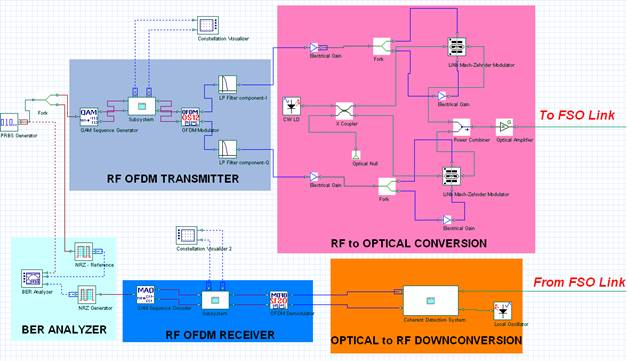Figure 1. The detailed structure of CO-OFDM FSO system via Optisystem interface.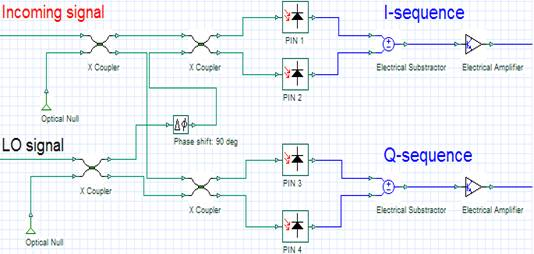Figure 2. The detailed structure of the coherent detection system via Optisystem interface.

3. Mathematical Analysis

3.1. OFDM Transmitter

Considering an OFDM system with N subcarriers, the complex baseband OFDM signal in the time domain s(t) can be expressed [5,7]: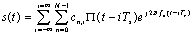(1)

where cn,i is the i-th information symbol of the n-th orthogonal subcarrier with frequency fn: ci=ai+jbi, where ai and bi are the set of amplitudes of the symbol, Ts is the symbol period, Π(t) is the unitary square pulse shaping function.

3.2. Optical Up-conversion

For the analysis of the optical I/Q modulator we use the widely accepted two tone model [5,10]. Two complex subcarriers tones at: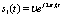(2)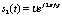(3)

where υ is the rms value of the subcarrier tone, are applied to the input of the I/Q modulator. Assuming that, the null bias condition for MZMs is optimal, the initial phase of the LD is φLD=0, the RF drive signals (VI,12, VQ,12) are relatively small and the nonlinearities are insignificant, we derive the expression for the resultant complex optical signal at the output of the optical modulator: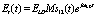(4)

where ELD is the average value of the electrical field of the LD transmitting radiation with angular frequency ωLD, the factor M is equal to υ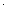π/2Vπ, Vπ is the half-wave switching voltage of each MZM and s12(t) is the produced complex signal by the mixed two tones: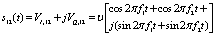(5)

This modulation technique results essentially in a linear replica of the signal s(t) up-converted to the center frequency of ωLD/2π. As a result, the transmitting optical power can be expressed: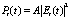(6)

where Α=cεο/2S. The c is the speed of light in air, εο is the dielectric constant of the air medium and S is the variable cross section of the gaussian profile laser beam through the path of the mobile FSO link.

The transmitted optical signal is suffered from atmospheric and scintillation fading due to weather conditions and atmospheric turbulence. The optical power of the signal according to the FSO transmission formula is: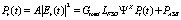(7)

where Gtotal is the total optical gain due to the amplifiers in each transceiver of the link terminals, LFSO is the FSO link calculation which combines attenuation and geometrical aspects based on the equation :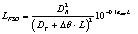(8)

where DR is the receive aperture diameter in m, DT is the transmit aperture diameter in m, Δθ is the beam divergence in mrad, L is the link range in km and αatm is the atmospheric attenuation factor in dB/km. The value of the factor αatm is calculated via the formula :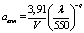(9)

where V is the visibility in km, λ is the wavelength of carrier in nm and q is a parameter evaluated between 0-1.6, according to weather conditions. The Ψ factor quantifies the variation of the signal fading due to atmospheric turbulence [3,9] and X has the value 1 or 0, in case of turbulence existence or not, respectively. Finally, PASE, is the overall power from Amplified Spontaneous Emission (ASE) due to the amplifier stages.

3.3.2. Turbulence Modelling

In our study, we use the Gamma-Gamma (GG) distribution as a turbulence model . According to GG distribution, that factorizes the irradiance I as the product of two independent random processes, the probability density function (pdf) of the variable I is [3,6,9,12]: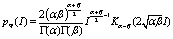(10)

where α and β are the effective numbers of small and large eddies, respectively,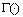is the gamma function and Kα-β(.) is the modified Bessel function of the second kind. The parameters α and β have the following form :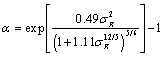(11)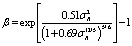(12)

where σR is Rytov variance which is proportional to refraction-index structure parameter cn2 (usually called as strength of turbulence):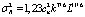(13)

where parameter k is the optical wavenumber, parameter cn2 is a function of altitude h and for computional purposes we take into account the Hufnagel-Valley (H/V) 21 model. According to this :(14)

where Α΄=1.7e-14m-2/3 and urms is the rms wind speed, urms=21m/s.

At the end of the link the transmitted optical power is detected and due to the down conversion procedures, the data are recovered. Assuming linearity in every stage of signal processing and ignoring the loss of the optical hybrid, the incoming signal and the radiation of the LO are mixed together to form coherent detection. For balanced detection, the noise term of each photodetector is completely cancelled, so that the complex photocurrent that consists in both I and Q components, can be written via the Er(t) and the conjugate complex of LO field, E*LO(t), as follows :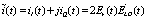(15)

3.5. BER Expressions

The BER expression for M-ary QAM is [13,14]:(16)

where γ1/2 is the known Q-factor equals to: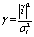(17)

where Βο is the optical ASE noise bandwidth used for OSNR measurements (typically 0.1nm), Rs is the symbol rate and σi is the total rms noise, without taking into account the noise that comes from background (sun radiation) and the ASE noises that occurs due to the amplifier stages. The σi expression that takes into consideration only the inherent detectors noises is given by the formula [2,15]: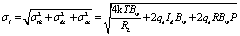(18)

where R is the PIN responsitivity, qe is the electron charge, h is the Planck constant, Bw is the bandwidth of the detector filters, k is the Boltzmann constant, T is the effective noise temperature, RL is the total effective input resistance of detectors, Id is the overall dark current of the photo- detectors and P is the total optical power that is detected through detection system.

4. Simulation Results & Discussion

Initially, we specify in the table I, the values of the project parameters.

Table I. Values of the parameters for the CO- OFDM system simulation.

 Block Component Parameters Value System PRBS generator BR 50Gbps OFDM TX-RX QAM generator M 4 OFDM N 512 Modulator NIFFT 1024 Demodulator Prefix points 0 Converter Up (Down) CW LD (LO) P 0dBm f 193.1THz Linewidth 0.05MHz Initial phase 0 LiNb-MZM Vπ 4V Amplifiers G 20dB NF 4dB FSO link Telescope TX-RX DT 5cm DR 20cm Δθ 2mrad Weather conditions V (aatm) 10km (0.1dB/km) 2km (0.99dB/km) 0.5km (7.82dB/km) Turbulence (X=1) h (cn2) 100m (6e-15m-2/3) 1000m (1e-16m-2/3) 5000m (1e-17m-2/3) Converter Down PIN photodiodes R 1A/W T 298K RL 50Ω Id 10nA Bw 50GHz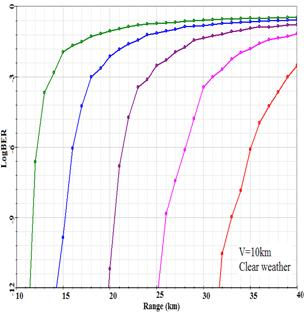Figure 3. LogBER vs Range for different number of subcarriers in clear weather conditions.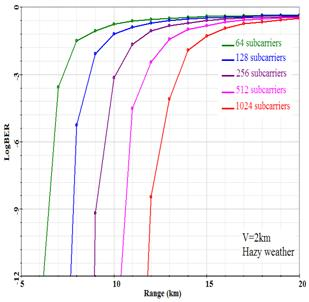Figure 4. LogBER vs Range for different number of subcarriers in hazy weather conditions.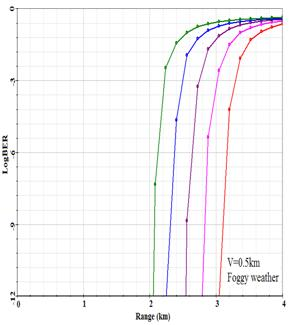Figure 5. LogBER vs Range for different number of subcarriers foggy weather conditions.

Fig. 3,4,5 illustrate the diagrams of LogBER vs Range for different numbers of subcarriers and different weather conditions. It is clear that the reliability of the link increases as visibility increases. It is also obvious that the larger number of subcarriers demonstrates better performance of the link. As the number of the subcarriers is increased, the quantity of the lost data is minimized, occurring at a non-ideality transmission channel, leading to decrease the bit error rate. To further explain, the maximum range that is achievable by the system (for a reliable link the corresponding BER value is equal to 1e-6) for clear sky conditions is 35km, for hazy weather is 12.5 km and for foggy weather is 3km. Fig. 6 shows that by decreasing the bitrate, the maximum achievable range for reliable link can be improved. Specifically, for hazy weather, N=512 subcarriers and for bitrates 20Gbps compare to 50Gbps and 100Gbps, more than 2Km and 4Km improvement could be obtained respectively.

Fig. 7 shows a BER vs OSNR diagram which indicates that higher bitrates are more sensitive to BER improvement as the OSNR increases. The OSNR measurements obtained with respect to broadband ASE noise in a fixed bandwidth equals to 0.1nm. It is evident that for bitrate equals to 20Gbps, there is no BER improvement. It could be interpreted as the system performance limit, caused by the increase of the signal transmitting power in order to improve the performance of the link.

Fig. 8 illustrates that the detected optical power is significant increased by higher QAM orders. It is worth mentioning that power difference between BPSK and 256-QAM is almost constant for any of the illustrated distances and equals to 12dB. Noteworthy that for any measurement we obtained, the noise had the same minimum value. In addition, the combination of higher received power and higher order constellation points does not guarantee the decrease of BER value, according to the expressions (16) and (17).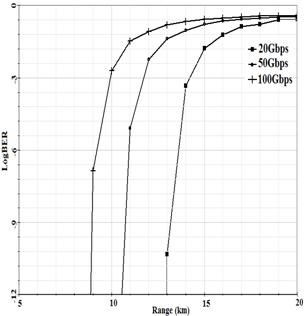Figure 6. LogBER vs Range for different Bitrates for V=2km.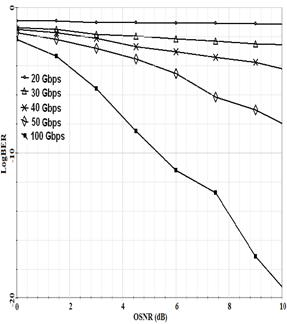Figure 7. LogBER vs Optical SNR for different Bitrates.

Fig. 9 presents the constellation diagrams for co-moving UAVs flying, with the same constant velocities, scenario. First of all, it is obvious that the transmission through the FSO link induced deviation to the constellation points in the I-Q plane, as it is shown in Fig. 9 (a)-(b). This was mainly due to the atmospherical fading that signal experienced through the horizontal path between the UAVs and all the other referred noise sources. Moreover, as the altitude of the UAVs decreases and the strength of turbulence increases, the adjacent points separation in the I-Q plane become less distinct. This indicates that the data errors raise and so the BER get a higher value. Indeed, at the altitude of 5000m, the diagram in Fig. 9 (c) appears to seem the same as the diagram refers to the transmission to the non turbulent link Fig.9 (b). This is based on the fact that as the altitude increases the turbulence effect becomes weaker, contrary to low heights, i.e. h=100m, as it is depicted in Fig.8 (e), showing that at these altitudes, the turbulence and scintillation effects play a major role to the link performance.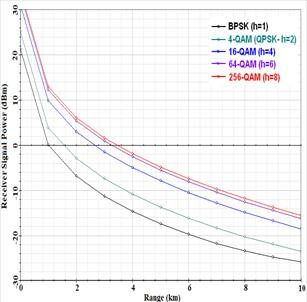Figure 8. Received power vs Range for various QAM flavours for V=2km.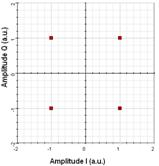(a)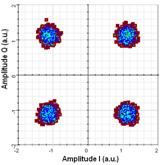(b)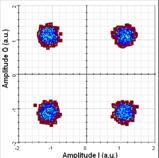(c)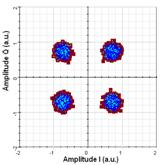(d)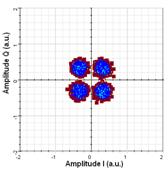(e)

Figure 9. The 4-QAM constellation diagrams in four different FSO link configurations between co-moving UAVs with fixed link range 4km and for three different altitudes above ground. (a) Before the transmission through the link. After the transmission through: (b) non turbulent link and turbulent link in (c) h=5000m, (d) h=1000m and (e) h=100m.

5. Conclusions

In this work, different configurations of a CO-OFDM FSO mobile system have been studied. The performance of the system has been evaluated for clear, hazy and foggy weather conditions and the results show that the system can provide a reliable communication link (BER value at 1e-6) for 35km, 12km and 3km respectively. It follows from this that as we increase the number of subcarriers and as we lower the bitrate, the performance of the system is improved. Also the system appears a sensitive behavior, changing the transmitting power. According to our results and considering various orders of QAM, it is approved that the higher order of QAM we introduce to the system, leads to significantly increase of the received power for specific transmitted power. Although this practice could provide a better SNR, it could not ensure a better BER. Based on the constellations points calculations, for higher order of QAM, as the constellation points increase, the separation distance among them shortens and as a result the data error probability gets higher. Thus, it is understood that turbulence effects which are more intense in low altitudes than in higher, tend to deteriorate the system performance. So, it is clear that the improvement of each subsystem is required in order to overcome any turbulence and scintillation effects, especially when the UAVs fly in low altitudes.

Acknowledgements

This research has been co-financed by the European Union (European Social Fund–ESF) and the Hellenic National Funds through the Operational Program "Education and Lifelong Learning" of the National Strategic Reference Framework (NSRF)-Research Funding Program: ARCHIMEDES III. Investing in knowledge society through the European Social Fund.

References

1. Antonis Hatziefremidis et al.: "Bit Error Rate Analysis along a Slanted Path Link Between UAVs and Ground Stations", in Proc. ICTON 2013, Cartaxena, Spain, June 2013.
2. Antonis Hatziefremidis et al.: "Flexible High Power Free Space Optical System for Aircraft to Ground Communications", in Proc. ICTON 2014, Austria, Graz, July 2014.
3. Abdelmoula Bekkali et al.: "Transmission Analysis of OFDM-Based Wireless Services Over Turbulent Radio-on-FSO Links Modeled by Gamma–Gamma Distribution",IEEE Photonics Journal, Vol.2, No.3, June 2010.
4. Neda Cvijetic et al.: "10Gb/s Free-Space Optical Transmission using OFDM", OFC Conference, 2008.
5. William Shieh and Ivan Djordjevic, "Orthogonal Frequency Division Multiplexing for Optical Communications",ELSEVIER, 2010.
6. Optisystem software version 13, technical support files.
7. Richard Van Nee and Ramjee Prasad, "OFDM for wireless multimedia communications", Artech House, 2000.
8. S. Bloom et al.: "Understanding the performance of free-space optics",Journal of Optical Networking, Vol.2, No.6, June 2003.
9. H.E. Nistazakis et al.: "BER estimation for multi-hop RoFSO QAM or PSK OFDM communication systems over gamma gamma or exponentially modeled turbulence channels", Optics&Laser Technology, 64 (2014)106–112.
10. Yan Tang et al.: "Optimum Design for RF-to-Optical Up-Converter in Coherent Optical OFDM Systems", IEEE Photonics Technology Letters, vol.19, No.7, April 2007.
11. Isaac I. Kim et al.: "Comparison of laser beam propagation at 785nm and 1550nm in fog and haze foroptical wireless communications",in SPIE Proceedings, vol.4214. pp. 26-37, Febr. 2001.
12. Nicolas Perlot: "Evaluation of the scintillation loss for optical communication systems with direct detection", Optical Engineering, Vol. 46(2), February 2007.
13. Arun K. Majumdar, Jennifer C. Ricklin: "Free-Space Laser Communications: Principles and Advances", Springer Publishing, 2008.
14. Wolfgang Freude et al: "Quality Metrics for Optical Signals: Eye Diagram, Q-factor, OSNR, EVM and BER", ICTON 2012, paper Mo.B1.5.
15. Pericles Tsekeris: "Optical Communications", University of Ioannina Publishings, 2003.

 Contents 1. 2. 3. 3.1. 3.2. 3.3. 3.4. 3.5. 4. 5.
Article ToolsAbstractPDF(402K)